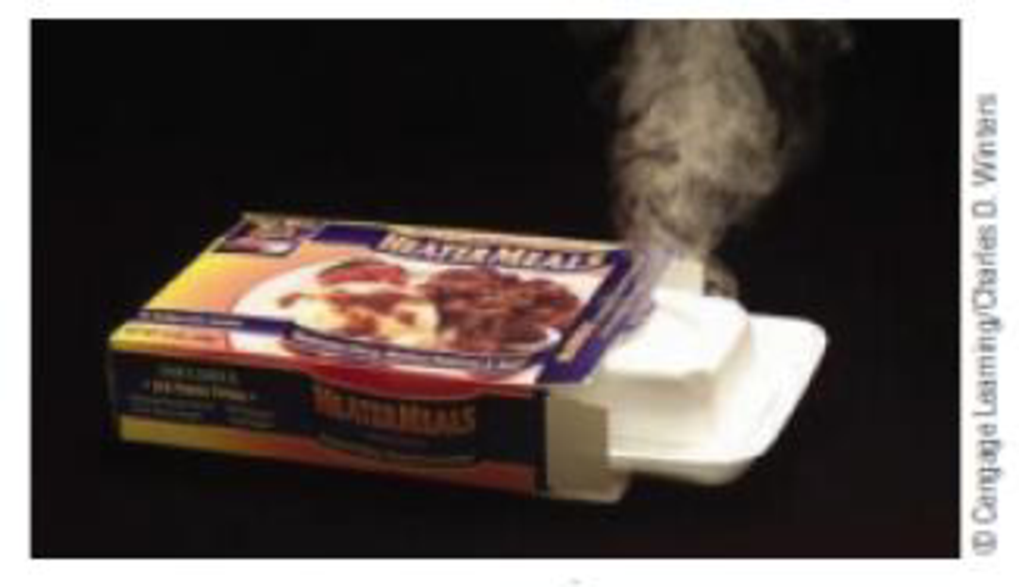# The meals-ready-to-eat (MREs) in the military can be heated on a flameless heater. You can purchase a similar product called “Heater Meals.” Just pour water into the heater unit, wait a few minutes, and you have a hot meal. The source of energy in the heater is Mg(s) + 2 H 2 O( ℓ ) → Mg(OH) 2 (OH) 2 (s) + H 2 (g) The “heater meal” uses the reaction of magnesium with water as a source of energy as heat. Calculate the enthalpy change under standard conditions, in joules, for this reaction. What quantity of magnesium is needed to supply the energy required to warm 25 mL of water ( d = 1.00 g/mL) from 25 °C to 85 °C? (See W. Jensen: Journal of Chemical Education, Vol. 77, pp. 713-717, 2000.)### Chemistry & Chemical Reactivity

9th Edition
John C. Kotz + 3 others
Publisher: Cengage Learning
ISBN: 9781133949640

#### Solutions

Chapter
Section### Chemistry & Chemical Reactivity

9th Edition
John C. Kotz + 3 others
Publisher: Cengage Learning
ISBN: 9781133949640
Chapter 5, Problem 99IL
Textbook Problem
651 views

## The meals-ready-to-eat (MREs) in the military can be heated on a flameless heater. You can purchase a similar product called “Heater Meals.” Just pour water into the heater unit, wait a few minutes, and you have a hot meal. The source of energy in the heater isMg(s) + 2 H2O(ℓ) → Mg(OH)2(OH)2(s) + H2(g)The “heater meal” uses the reaction of magnesium with water as a source of energy as heat.Calculate the enthalpy change under standard conditions, in joules, for this reaction. What quantity of magnesium is needed to supply the energy required to warm 25 mL of water (d = 1.00 g/mL) from 25 °C to 85 °C? (See W. Jensen: Journal of Chemical Education, Vol. 77, pp. 713-717, 2000.)

Interpretation Introduction

Interpretation:

The enthalpy change and quantity of Mg needed has to be calculated.

Concept Introduction:

Standard enthalpy of the reaction,ΔrHo, is the change in enthalpy that happens when matter is transformed by a given chemical reaction, when all reactants and products are in their standard states. Enthalpy of the reaction,ΔrH, is the change in enthalpy that happens when matter is transformed by a given chemical reaction

Heat energy required to raise the temperature of 1g of substance by 1K..Energy gained or lost can be calculated using the below equation.

q=C×m×ΔT

Where, q= energy gained or lost for a given mass of substance (m), C =specific heat capacity,ΔT= change in temperature.

The standard enthalpy change of combustion of a compound is the enthalpy change which occurs when one gram of the compound is burned completely in oxygen under standard conditions, and with everything in its standard state.

ΔrH0=ΣnΔfH0(products)-ΣnΔfH0(reactants)

### Explanation of Solution

Given,

ΔfH Of Mg=0

ΔfH Of water =-285.83kJ/mol

ΔfH Of Mg(OH)2=-924.54kJ/mol

ΔfH Of H2=0

ΔrH0=ΣnΔfH0(products)-ΣnΔfH0(reactants)

ΔrH= 0+(1mol×-924.54kJ/mol)- 0+(2mol×-285.83kJ/mol)

ΔrH=-352

### Still sussing out bartleby?

Check out a sample textbook solution.

See a sample solution

#### The Solution to Your Study Problems

Bartleby provides explanations to thousands of textbook problems written by our experts, many with advanced degrees!

Get Started